Courses

# Chapter Notes - Cost Of Production, Class 12, Economics | EduRev Notes

## Commerce : Chapter Notes - Cost Of Production, Class 12, Economics | EduRev Notes

The document Chapter Notes - Cost Of Production, Class 12, Economics | EduRev Notes is a part of the Commerce Course Economics Class 11.
All you need of Commerce at this link: Commerce

COST FUNCTION :: It studies the FUNCTIONAL RELATIONSHIP between output and cost of production. It tells the least cost combination of inputs for different levels of output C = f (Q)

MEANING OF COST :: Ordinary, money expenditure incurred by a firm in production of a commodity is called the cost of production. A firm requires factor inputs  and non-factor inputs for producing a commodity and the firm pays them in the form of money e.g rent to the landlord, salaries and wages to the labour, interest to the capitalist and expenditure on other inputs like raw material, power, transportation, Insurance charges, etc.
These are costs to the firm and are called money costs because these are paid in the form of money.

 ⇒ But in economics, the concept ‘cost’ is used in a broader sense.In economics sum ofexplicit costs(accounting) and implicit costs (non-accounting)  constitute the total cost of production.

In SHORT PERIOD, costs can be categorised into fixed cost and variable cost, but in long period all cost are variable cost

 B.O.D TOTAL FIXED COST (TFC) TOTAL VARIABLE COST (TVC) MEANING These are those cost which donot changes with change in output i.e they remains constant at all level of output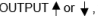fixed cost remains constant. These are those cost which changes with change in output .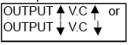INCURRED ON These are incurred on Fixed factor of production These are incurred on Variable factor of production KNOWN AS These cost are also known as→ Supplement Cost    or → Overhead Cost      or → Indirect Cost            or → General Cost             or → Unavoidable cost         or These cost are also known as→ Prime  Cost  or → Direct Cost   or → Avoidable Cost EXAMPLE Examples are Rent, Insurance premium , Cost of P & M,  Licence fee , Wages and Salaries of permanent staff Examples : Raw material like cotton for shirt, leather for shoes , Wages and Salary,Electricity , Fuel expenses SITUATION OF ZERO Fxed cost can never be zero. It is positive even at zero level of output Such cost are incurred till there is production and hence it becomes zero at zero level of output CONTINU- ANCE Production can be carried at the loss of fixed cost. Fixed cost is maximum loss a producer can suffer (borne) A producer must recover variable cost otherwise he will stop production SHAPE T.F.C curve is PARALLEL TO X-AXIS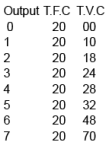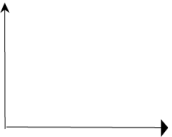It starts from origin and is  INVERSE S - SHAPED that is→ Initially increases at a diminishingrate (due to increasing return to factor)→  Then increases at a constant rate(due to constant return to factor)  →Finally increases at increasingrate (due to decreasing return to factor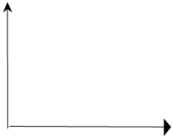TOTAL COST  :: It is all the expenditure incurred in production of a commodity. It is summation of Total Fixed cost (TFC)  and Total Variable Cost (TVC)

 TC = TFC + TVC TC =  ATC x Output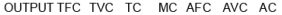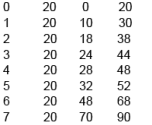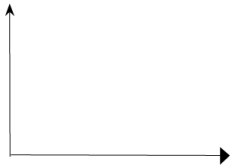BEHAVIOUR OF TOTAL COST

(1) ZERO LEVEL OF OUTPUT :: At zero level of output Total fixed cost (TFC) is equal to Total Cost (TC) which means that total cost is positive even at zero level of output and thus TC curve starts from the point as intercept on Y-axis.

(2) PARALLEL TO TVC :: The vertical distance between Total Cost (TC) and Total Variable Cost (TVC) on a graph is Total Fixed Cost (TFC) [ as TC - TVC = TFC].

→ Since TFC is constant at all levels of output ,therefore the vertical distancebetween Total cost and Total variable cost will also be same which means that both becomes parallel lines.Thus The change in TC is entirely due to TVC as TFC is constant at all level of output.

(3) SHAPE :: Starts from intercept on Y-axis and is Inverse S-shaped. It means TC curve starts from origin and

→  Initially increases at a diminishing rate
→  Then increases at a constant rate
→  Finally increases at increasing rate

MARGINAL COST :: It is ADDITIONAL COST incurred for PRODUCTION OF ADDITIONAL UNIT by  employing additional factor of production.

(1) MC CURVE IS U - SHAPED which means this cost initially decreases with increase in output but after reaching a certain point the cost starts increasing.

(2) MC is NOT AFFECTED BY FIXED COST as fixed cost remains constant for all level of output and can never be additional cost. Thus MC is affected by variable cost only that changes with change in output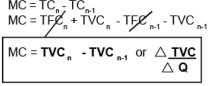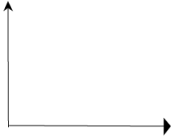RELATION BETWEEN TVC AND MC

MC refers to additional cost and additional cost can only be variable cost. Accordingly sum total of Marginal Cost at all level of output will be total variable cost and not total cost because total cost includes fixed cost i.e

MC = TVCn   - TVCn-1

 Σ MC = TVC and Σ MC ≠ TC .GRAPHICALLY

→ Up to point Q*, TVC is increasing at a decreasing rate,because MC is decreasing.
→ Beyond point Q*, TVC is increasing at an increasing rate,because MC is increasing.
→ At point Q*, TVC stops increasing at a decreasing rate,because MC touches its lowest point.

It means that area under MC at any level of output isTotal variable cost (TVC). Thus if OB level of output is produced area OBCD will be equal to total variable cost

Consider any level of output say six units at this level TVC = 48 which is summation of MCAVERAGE CONCEPTS

(1) AVERAGE FIXED COST (AFC) :: It is PER UNIT FIXED COST of producing goods.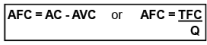(2) AVERAGE VARIABLE COST (AVC) :: It is PER UNIT VARIABLE COST of producing goods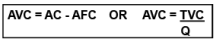SHAPE :: U - Shaped

(3) AVERAGE TOTAL COST (ATC) or AVERAGE COST (AC) :: It is PER UNIT TOTAL COST of producing goods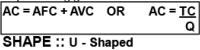AFC’S NATURE // PROPERTIES // CHARACTERISTIC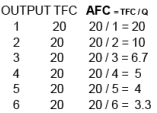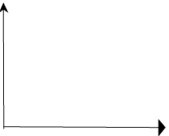(1) AVERAGE FIXED COST (AFC) GOES ON DECREASING WITH INCREASE IN OUTPUT :: AFC is total fixed cost (TFC) divided by output and as the output is increased , the constant fixed cost gets distributed to increased number of output and this cause
Average fixed Cost (AFC) to decrease.
As depicted in the example as output is increased from 1unit to 2 unit the TFC (=20) gets distributed to 2 units and AFC becomes Rs10/- per unit

(2) AFC IS NEVER ZERO Therefore it never touches X-axis

(3) It assumes the SHAPE OF RECTANGULAR HYPERBOLA which means at any level of output area under the curve is same.
Area under the AFC curve represents AFC x Q which equals Total fixed Cost (TFC) and as we know TFC remains constant for all level of output , therefore Area at all points on rectangular hyperbola is same

At OB level of output ,  Area = OB x OC = 2 x 10 = 20
At OD level of output ,  Area =  OD x OE = 4 x 5  = 20

RELATIONSHIP BETWEEN AVERAGE TOTAL COST (ATC) WITH MARGINAL COST (MC)

Note :: It is MC which brings about changes (rise or fall) in AC and not the other way round.

(1) Both are derived from Total Cost as Ac = TC/Q and  MC = TCN - TCN-1

(2) W hen AC is falling , MC is below it (as MC declines faster than AC)

 AC > MC

(3) W hen AC is rising , MC is above it (as MC rises faster than AC)

 AC < MC

(4) MC cuts AC at AC’s minimum point i.e when AC is constant

 AC = MC

(5) MC CAN RISE WHEN AC IS FALLING  :: Since average cost is related to many or all  units of output so any increase or decrease in cost is distributed to all the units whereas in case of MC any change in cost is borne by additional unit only which makes MC change faster than AC. Hence MC  decreases faster than AC and reaches its minimum point  “A” earlier than minimum point of AC (point “B”). MC starts rising thereafter (from point A to B) whereas AC continues to decrease (from point C to B)

The relationship between marginal cost and average cost is an arithmetic relationship. To understand  this relationship let us take a numerical example.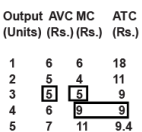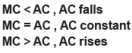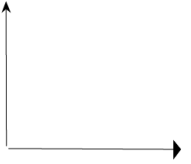CAN AC RISE WHEN MC IS FALLING ?  NO, because when MC falls , AC will also fall.

 SPECIAL POINT :: The relationship between marginal cost (MC) and  average variable cost (AVC) is similar to the relationship between  marginal cost and average cost because marginal cost is not affected by fixed cost.Thus, thi s relationship between marginal cost and average cost is a generalized relationship and holds valid in case of the marginal and average values of any variable, be it revenue or product etc.

SHOW AC (ATC), AVC, MC, AFC ON A SINGLE DIAGRAM

 (1) AFC falls, approaches X - axis but never touches it and thus assumes the shape of RECTANGULAR HYPERBOLA(2) AC, AVC, MC CURVES ARE U - SHAPED that is these cost curves initially decreases with increase in output but later on rises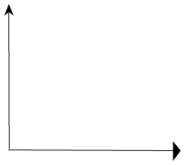AC AND AVC

(1) AC CURVE WILL ALWAYS LIE ABOVE THE AVC CURVE because AC includes both AVC and AFC at all levels of output and thus is greater than AVC by amount of AFC (AC - AVC = AFC)

(2) AVC REACHES ITS MINIMUM POINT (POINT “C”) AT A LEVEL OF OUTPUT LOWER THAT THAT OF AC ( POINT “B”) because when AVC is at its minimum point , AC is still falling because of falling AFC. Thus minimum of AC is to the right of minimum point of AVC

(3) With increase in output , the GAP/ VERTICAL DSITANCE  BETWEEN AC AND AVC DECREASE because the gap is AFC which coninues to decline with rise in output

(4) AC AND AVC NEVER INTERSECT each other because AFC can never be zero

MC WITH AC AND AVC

(1) WHEN MC IS LESS THAN AC & AVC , both of them FALL with increase in output that is

 (AC or AVC) > MC

(2) W hen MC becomes equal to AC and AVC , they become constant. MC curve cuts AC curve at Point “B” and AVC at point “C” at their minimum points that is

 (AC or AVC) = MC

(3) When MC IS MORE THAN AC AND AVC , both of them RISES with increase in output that is

 MC > (AC or AVC)

 SPECIAL POINT(a) MC = AVC = TVC at first level of output (b) Minimum point of MC comes before the minimum points of AC and AVC curves(c) MC curve cuts both AC and AVC curves at their minimum points

WHAT IS U-SHAPED CURVE ? WHY AC, AVC AND MC ARE U-SHAPED ?

U- shaped cost curve means a curve that initially cost decreases with increase in output and after reaching a certain point (minimum point) it starts rising due to following reason

(1) APPLICATION OF LAW OF VARIABLE PROPORTION :: According to this law when in short run additional variable input (like raw material, labour) is employed on fixed factors, then
- initially increasing return sets in which causes proportional more increase in output than input and hence cost decreases,
- But after a certain output decreasing return prevails which means proportional less increases in output and hence cost increases.

(2) DUE TO UTILIZATION OF FIXED FACTORS :: Fixed cost on fixed factors is constant and hence when more output is produced by fully utilizing fixed factors, the same fixed cost gets distributed to large number of units produced which reduces per unit and marginal cost. However, later on frequent breakdown of fixed factors occurs due to over utilisation which causes repair and maintenance cost as well as overall cost to increase.

(3) AC IS VERTICAL SUMMATION OF AVC AND AFC :: In earlier stages both AVC and AFC decreases, these cause AC to decrease. After a certain output AVC increases and this increase is more powerful than decrease in AFC, thus AC starts rising

DIFFERENCE BETWEEN EXPLICIT (ACCOUNTING) COST AND IMPLICIT (NON ACCOUNTING) COST

 B.O.D EXPLICIT COST  or ACCOUNTING COST IMPLICIT COST  or NON ACCOUNTING COST MEANING It refers to actual money expenditure of a firm in(i) purchasing non- factor input and(ii) hiring factor input (L,L,C,E) Implicit cost are cost of self owned and self employed factors Entry in books of account These cost are recorded in books of account as it involves actual expenditure on buying and hiring These cost are not recorded in books of account as no money cost is involved Well defined or not These cost are well defined These cost are not well defined and hence are calculated (imputed cost) contractual payment or notExample These cost are generally contractual payment and are made to outsidersWages paid to employer , Rent , Interest paid to bank These cost are not made to outsidersInterest  on own capital, rent on own land, Salaries for own labour

EXAMPLE :: Leaving a job of Rs. 40,000 per month and using his factory evicting where tenant was paying Rs. 50,000 per month, an entrepreneur undertakes production of garments. He pays Rs. 1,00,000 for the purchase of inputs. Find cost of production in terms of explicit cost and implicit cost.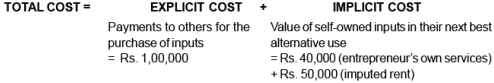Total Cost = Rs. 1,00,000 + Rs. 90,000  = Rs. 1,90,000

HOW TO MEASURE IMPLICIT COST :: Implicit cost is measured by determining the value of self occupied factors in terms of their market price.

Eg : if a doctor has opened a clinic at his home, then imputed value of salary for his service will be calculated on the basis of salary that he would receive if he is employed by someone else

FORMULA’S AT A GLANCE

 TFC(1)  Equal to TC at zero level(2)  AFC X Output (3)  TC - TVC TVC(1)  equal to summation of MC i.e TVC = Σ MC(2) AVC X Output(3) TC - TFC TC(1) MC + TFC(2)  ATC X Output(3) TFC + TVC

 AFC(1) TFC  /  Output(2) ATC - AVC AVC(1) TVC  /  Output(2) ATC - AFC AC(1) TC  / Output(2) AVC + AVC MC(1) TCn - TCn-1(2) TVCn - TVCn-1(3)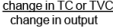Offer running on EduRev: Apply code STAYHOME200 to get INR 200 off on our premium plan EduRev Infinity!

## Economics Class 11

207 videos|195 docs|64 tests

,

,

,

,

,

,

,

,

,

,

,

,

,

,

,

,

,

,

,

,

,

,

,

,

,

,

,

;